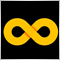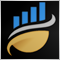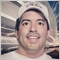# NormaizeDouble() floating point problem?36377

Hi, im trying to find a way around the problem of floating point numbers when trying to use it as a stoploss

In the following :

`double cAsk = MarketInfo(_Symbol,MODE_ASK);`

Sometimes cAsk becomes a floating point number EG 0.8626200000000001

Im having real trouble trying to use this number as a stoploss value and is giving me error 130

When i use NormalizeDouble() as follows it doesnt work

```double cBid = MarketInfo(_Symbol,MODE_BID);
double newBid=NormalizeDouble(cBid,_Digits);```

I then try to turn the double to string

```string newBidStr = DoubleToStr(cBid,_Digits);
double newBidDb = StrToDouble(newBidStr);
```

This works as a string but how can i use a string as a double?

When i change it back to double it sometimes goes back to a long floating point number EG 0.8626200000000001

I do say sometimes. Not always sometimes it works sometimes its still a problem?

Not sure what to do?51043

Stephen Reynolds:

Hi, im trying to find a way around the problem of floating point numbers when trying to use it as a stoploss

In the following :

Sometimes cAsk becomes a floating point number EG 0.8626200000000001

Im having real trouble trying to use this number as a stoploss value and is giving me error 130

When i use NormalizeDouble() as follows it doesnt work

I then try to turn the double to string

This works as a string but how can i use a string as a double?

When i change it back to double it sometimes goes back to a long floating point number EG 0.8626200000000001

I do say sometimes. Not always sometimes it works sometimes its still a problem?

Not sure what to do?

`NormalizeDouble(StringToDouble(newBidStr),_Digits)`25333

Stephen Reynolds: Sometimes cAsk becomes a floating point number EG 0.8626200000000001
Nikolaos Pantzos:
`NormalizeDouble(StringToDouble(newBidStr),_Digits`
You used NormalizeDouble, It's use is usually wrong, as it is in your case.
1. Floating point has a infinite number of decimals, it's your not understanding floating point and that some numbers can't be represented exactly. (like 1/10.)
Double-precision floating-point format - Wikipedia, the free encyclopedia

2. Print out your values to the precision you want with DoubleToString - Conversion Functions - MQL4 Reference.

3. SL/TP (stops) need to be normalized to tick size (not Point) — code fails on non-currencies. (On 5Digit Broker Stops are only allowed to be placed on full pip values. How to find out in mql? - MQL4 programming forum) and abide by the limits Requirements and Limitations in Making Trades - Appendixes - MQL4 Tutorial and that requires understanding floating point equality Can price != price ? - MQL4 programming forum

4. Open price for pending orders need to be adjusted. On Currencies, Point == TickSize, so you will get the same answer, but it won't work on non-currencies. So do it right: Trailing Bar Entry EA - MQL4 programming forum or Bid/Ask: (No Need) to use NormalizeDouble in OrderSend - MQL4 programming forum

5. Lot size must also be adjusted to a multiple of LotStep and check against min and max. If that is not a power of 1/10 then NormalizeDouble is wrong. Do it right.

6. MathRound() and NormalizeDouble() are rounding in a different way. Make it explicit.
MT4:NormalizeDouble - MQL5 programming forum
How to Normalize - Expert Advisors and Automated Trading - MQL5 programming forum

7. Prices you get from the terminal are already normalized.

8. PIP, Point, or Tick are all different in general.
What is a TICK? - MQL4 programming forum 2014.08.03371

Stephen Reynolds:

Hi, im trying to find a way around the problem of floating point numbers when trying to use it as a stoploss

In the following :

Sometimes cAsk becomes a floating point number EG 0.8626200000000001

Im having real trouble trying to use this number as a stoploss value and is giving me error 130

Not sure what to do?

```double cAsk = NormalizeDouble( MarketInfo(_Symbol,MODE_ASK), (int)MarketInfo(_Symbol,MODE_DIGITS));